﻿ 调水工程对调蓄水库水温影响的研究 Effect of Water Transfer Project on Regulating Reservoir Water Temperature

Journal of Water Resources Research
Vol.07 No.02(2018), Article ID:23960,9 pages
10.12677/JWRR.2018.72014

Effect of Water Transfer Project on Regulating Reservoir Water Temperature

Yanling Yao1, Xinzhou Zhang2, Jun Lv1, Xiang Li3

1Songliao River Water Environmental Science Research Institute, Songliao River Basin Water Resources Protection Bureau, Changchun Jilin

2Key Lab of Port, Waterway and Sedimentation Engineering of the Ministry of Transport, Nanjing Hydraulic Research Institute, Nanjing Jiangsu

3Department of Port and Coastal Engineering, Hohai University, Nanjing Jiangsu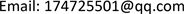Received: Feb. 12th, 2018; accepted: Feb. 24th, 2018; published: Mar. 1st, 2018ABSTRACT

Water transfer project is the necessary engineering measure to solve the problem of insufficient water resources or the imbalanced spatial and temporal distribution problems. Water diversion between different reservoirs through the pipeline and river will affect the reservoir water temperature. The two-dimensional hydrodynamic-thermal mathematical model is used to simulate the influence of the Jilin Province Water Diversion Project on the water temperature of Shitoukoumen Reservoir. The results show that the effect of the project to the range and time of the reservoir water temperature regulation is closely related to the amount of water transferred, the water temperature difference, the water depth of the reservoir and the position of the water inlet. The water amount of Jilin Province Water Diversion Project is very small compared with that of the storage reservoirs, and the influence of water diversion on the water temperature distribution in the reservoir is limited. The water diversion inlet is located at the end of the reservoir. The affected area is basically limited to the local area of the reservoir tail. The impact of water temperature reaches equilibrium within one month, with little impact on the whole reservoir area and the dam. Water diversion project away from the dam site can reduce the impact of the reservoir and downstream rivers.

Keywords:Water Diversion Project, Reservoir, Water Temperature Influence, Mathematical Model

1松辽流域水资源保护局松辽水环境科学研究所，吉林 长春

2南京水利科学研究院港口航道泥沙工程交通行业重点实验室，江苏 南京

3河海大学港口海岸与近海工程学院，江苏 南京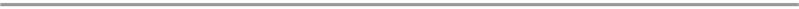1. 引言

20世纪60年代初美国最先开始研究水库水温的分布和变化规律。此后，许多学者采用不同种方法和手段对水库库区水温分布进行大量研究工作，取得了许多有意义的成果。如刘平等  通过经验公式法对迪那河五一水库水温及下泄水温进行了预测分析。脱友才、杨红秀分别对丰满水库和汾河水库不同季节的水温变化分布规律进行了原型观测和资料分析   。赵一慧、甘衍军等   通过EFDC数学模型模拟了小浪底、二滩水库的水温分布变化规律。江春波、戴凌全等   对水库水温数学模型研究进展进行了综述，指出了不同模型的优缺点以及需要进一步研究的方向。李林等  通过三维水温模型研究了支流影响下的水库水温变化特征，表明支流入流越靠近坝址对坝前水温分布影响越大。高学平、柳海涛等   通过水温模型试验模拟了糯扎渡水库等大型水库的水温分布规律。

2. 工程概况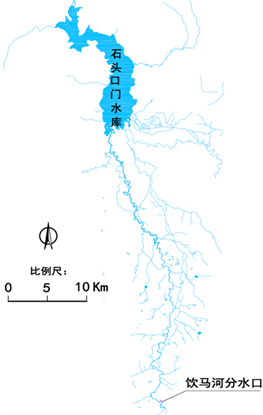Figure 1. Sketchmap of the Yinma River basin and Shitoukoumen reservoir

3. 数学模型的建立

3.1. 水流基本方程

1) 连续方程

$\frac{\partial Z}{\partial t}+\frac{\partial Hu}{\partial x}\frac{\partial Hv}{\partial y}=q$ (1)

2) 动量方程

$\frac{\partial Hu}{\partial t}+\frac{\partial Huu}{\partial x}+\frac{\partial Huv}{\partial y}=-gH\frac{\partial Z}{\partial x}-\frac{\partial H\stackrel{¯}{{u}^{\prime }{u}^{\prime }}}{\partial x}-\frac{\partial H\stackrel{¯}{{u}^{\prime }{v}^{\prime }}}{\partial y}+fHv+\frac{{\tau }_{sx}-{\tau }_{bx}}{\rho }+q{u}_{*}$ (2)

$\frac{\partial Hv}{\partial t}+\frac{\partial Huv}{\partial x}+\frac{\partial Hvv}{\partial y}=-gH\frac{\partial Z}{\partial y}-\frac{\partial H\stackrel{¯}{{v}^{\prime }{u}^{\prime }}}{\partial x}-\frac{\partial H\stackrel{¯}{{v}^{\prime }{v}^{\prime }}}{\partial y}-fHu+\frac{{\tau }_{sy}-{\tau }_{by}}{\rho }+q{v}_{*}$ (3)

3.2. 二维温水扩散运动方程

$\frac{\partial \Delta TH}{\partial t}+\frac{\partial \left(Hu\Delta T\right)}{\partial x}+\frac{\partial \left(Hv\Delta T\right)}{\partial y}=D{}_{x}H\frac{{\partial }^{2}\Delta T}{\partial {x}^{2}}+{D}_{y}H\frac{{\partial }^{2}\Delta T}{\partial {y}^{2}}-\frac{k\Delta T}{\rho {C}_{P}}+q\Delta {T}_{i}$ (4)

3.3. 求解方法及定解条件

1) 求解方法

2) 初始条件

3) 边界条件

$\frac{\partial \left(D\Delta T\right)}{\partial t}+\frac{1}{{C}_{\xi }{C}_{\eta }}\left[\frac{\partial }{\partial \xi }\left(D\Delta Tu{C}_{\eta }\right)+\frac{\partial }{\partial \eta }\left(D\Delta Tv{C}_{\xi }\right)\right]=0$ (5)

$\Delta T|{}_{{\Gamma }_{0}}=\Delta {T}_{a}\left(t,\xi ,\eta \right)$ (6)

4) 动边界

3.4. 计算条件

3.5. 计算参数

4. 调水影响分析

4.1. 监测点水温变化

4.2. 调水水温影响范围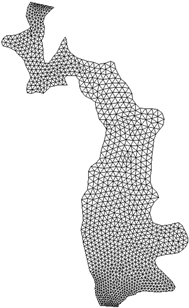Figure 2. Computational grid of reservoir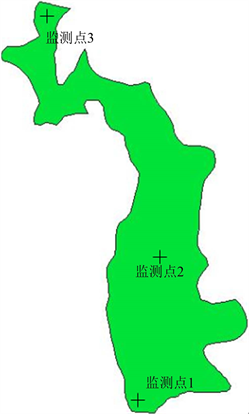Figure 3. The layout of observation point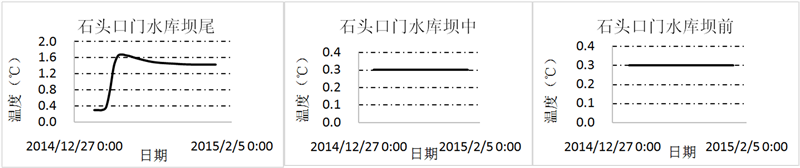Figure 4. Change of water temperature at observation point in January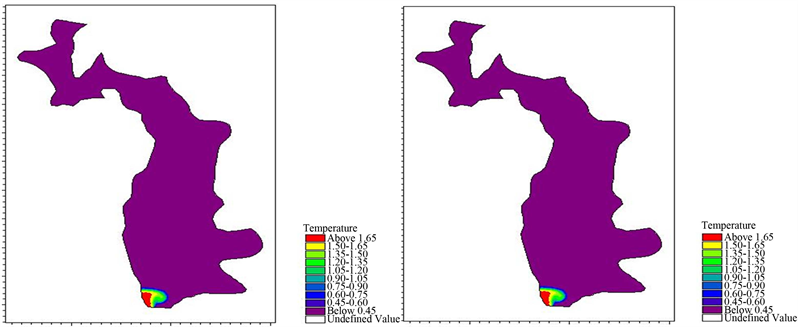5 d 15 d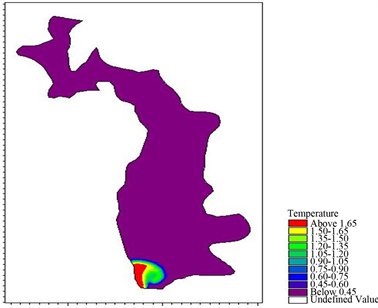30 d

Figure 5. Variation distribution of water temperature in 30 days in JanuaryTable 1. Water temperature change at observation pointTable 2. Influence distance of water temperature change

4.3. 调水水温影响距离

5. 结论

1) 调水对水库水温影响的范围、时间主要和调水量、调水温差、水库水深、入水口位置等有密切关系。

2) 由于调水量和水库水量相比并不大，调水对水库水温分布的影响有限，受影响的范围基本局限于库末局部范围内，水温影响在1个月内即达到平衡。

3) 调水工程入水口位置尽量设置在水库库尾，远离坝址可以防止调水对水库坝前以及下游河流产生影响。

4) 平面二维水流水温数学模型可以对水库调水影响进行合理的模拟预报。但水库水深相对较大时，水温和水库水体的掺混具有典型的三维特征，更精细的预报需要借助于三维数学模型来模拟。

Effect of Water Transfer Project on Regulating Reservoir Water Temperature[J]. 水资源研究, 2018, 07(02): 126-134. https://doi.org/10.12677/JWRR.2018.72014

1. 1. 汪明娜, 汪达. 调水工程对环境利弊影响综合分析[J]. 水资源保护, 2002(4): 10-14. WANG Mingna, WANG Da. Comprehensive analysis of environmental advantages and disadvantages of water diversion project. Water Resources Protection, 2002(4): 10-14. (in Chinese)

2. 2. 张军锋, 闫莉, 崔玉香, 等. 引大济湟调水总干渠工程生态环境影响[J]. 水资源保护, 2011(9): 88-91. ZHANG Junfeng, YAN Li, CUI Yuxiang, et al. The ecological environmental impact of the diversion of the water diversion project in the main canal of the diversion project. Water Resources Protection, 2011(9): 88-91. (in Chinese)

3. 3. 刘莹, 王冬梅, 刘孝盈, 等. 国外调水工程对生态与环境影响的研究与对策[J]. 中国水利, 2008(8): 64-68. LIU Ying, WANG Dongmei, LIU Xiaoying, et al. Research and countermeasures on ecological and environmental impact of water diversion project in foreign countries. China Water Conservancy, 2008(8): 64-68. (in Chinese)

4. 4. 赵敏, 常玉苗. 跨流域调水对生态环境的影响及其评价研究综述[J]. 水利经济, 2009, 27(1): 1-4. ZHAO Min, CHANG Yumiao. The impact of water diversion on the ecological environment and the evaluation of the evaluation. Water Conservancy Economy, 2009, 27(1): 1-4. (in Chinese)

5. 5. 刘建康, 曹文宣. 长江流域的鱼类资源及其保护对策[J]. 长江流域资源与环境, 1992, 1(1): 17-23. LIU Jiankang, CAO Wenxuan. Fish resources in the Yangtze river basin and their protection countermeasures. Resources and Environment in the Yangtze River Basin, 1992, 1(1): 17-23. (in Chinese)

6. 6. 蒋固政. 长江流域大型水利工程与鱼类资源救护[J]. 人民长江, 2008, 39(23): 62-64. JIANG Guzheng. Large water conservancy project and rescue of fish resources in Yangtze river basin. People’s Yangtze River, 2008, 39(23): 62-64. (in Chinese)

7. 7. 韩彩霞, 尹晓煜, 王伟, 等. 预测吴家庄水库下泄水温对农作物的影响[J]. 水利科技与经济, 2002, 8(3): 155-156. HAN Caixia, YIN Xiaoyu, WANG Wei, et al. Predict the effect of water temperature on the lower water temperature of Wujiazhuang Reservoir. Water Conservancy Technology and Economy, 2002, 8(3): 155-156. (in Chinese)

8. 8. 吴佳鹏, 黄玉胜, 陈凯麒. 水库低温水灌溉对小麦生长的影响评价[J]. 中国农村水利水电, 2008(3): 68-71. WU Jiapeng, HUANG Yusheng and CHEN Kaiqi. Evaluation of the impact of low temperature water irrigation on wheat growth. China Rural Water and Hydropower, 2008(3): 68-71. (in Chinese)

9. 9. 刘平, 张岩. 迪那河五一水库水温预测分析研究[J]. 吉林水利, 2010(7): 27-30. LIU Ping, ZHANG Yan. Analysis of the water temperature prediction of Wuyi Reservoir in Dadi River. Jilin Water Conservancy, 2010(7): 27-30. (in Chinese)

10. 10. 脱友才, 刘志国, 邓云, 等. 丰满水库水温的原型观测及分析[J]. 水科学进展, 2014, 25(5): 731-738. TUO Youcai, LIU Zhiguo, DENG Yun, et al. The prototype observation and analysis of the water temperature of the fullness reservoir. Progress of Water Science, 2014, 25(5): 731-738. (in Chinese)

11. 11. 杨红秀. 汾河水库水温分布的现场试验[J]. 华北水利水电大学学报(自然科学版), 2010, 31(2): 6-8. YANG Hongxiu. Field test of water temperature distribution in Fenhe Reservoir. Journal of North China Water and Hydro-power University (Natural Science Edition), 2010, 31(2): 6-8. (in Chinese)

12. 12. 赵一慧, 逄勇. 基于EFDC模型的小浪底水库水温研究[J], 四川环境, 2017(4): 24-31. ZHAO Yihui, PANG Yong. Study on the water temperature of Xiaolangdi Reservoir based on EFDC model. Sichuan Envi-ronment, 2017(4): 24-31. (in Chinese)

13. 13. 甘衍军, 李兰, 武见, 等. 基于EFDC的二滩水库水温模拟及水温分层影响研究[J]. 长江流域资源与环境, 2013, 22(4): 476. GAN Yanjun, LI Lan, WU Jian, et al. The study on the influence of water temperature simulation and water temperature stratification in Ertan Reservoir based on EFDC. Resources and Environment of Yangtze River Basin, 2013, 22(4): 476. (in Chinese)

14. 14. 江春波, 周琦, 刘健. 河道与水库水温模拟及预测模型概述[J]. 环境影响评价, 2016, 38(3): 53-56. JIANG Chunbo, ZHOU Qi and LIU Jian. Overview of water temperature simulation and prediction model of river and reservoir. Environmental Impact Assessment, 2016, 38(3): 53-56. (in Chinese)

15. 15. 戴凌全, 戴会超, 王煜. 水库水温数学模型研究综述[J]. 三峡大学学报(自然科学版), 2010, 32(4): 6-10. DAI Lingquan, DAI Huichao and WANG Yu. A review of mathematical models of water temperature in reservoirs. Journal of the Three Gorges University (Natural Science Edition), 2010, 32(4): 6-10. (in Chinese)

16. 16. 高学平, 陈弘, 王鳌然, 等. 糯扎渡水电站多层进水口下泄水温试验研究[J]. 水力发电学报, 2010, 29(3): 126-131. GAO Xueping, CHEN Hong, WANG Aoran, et al. The experimental study on the water temperature of multi-layer intake of Wazundu Hydropower Station. Journal of Hydroelectricity, 2010, 29(3): 126-131. (in Chinese)

17. 17. 柳海涛, 孙双科, 王晓松, 等. 大型深水库分层取水水温模型试验研究[J]. 水力发电学报, 2012, 31(1): 129-134. LIU Haitao, SUN Shuangke, WANG Xiaosong, et al. Experimental study on the water temperature model of water intake in large deep reservoirs. Journal of Hydroelectricity, 2012, 31(1): 129-134. (in Chinese)

18. 18. 李林, 周孝德, 宋策. 基于三维水温模型预测有支流影响下的水库水温[J]. 水土保持通报, 2012, 32(2): 159-161. LI Lin, ZHOU Xiaode and SONG Ce. Based on the three-dimensional water temperature model to predict the water temperature of the reservoir under the influence of tributaries. Water and Soil Conservation Bulletin, 2012, 32(2): 159-161. (in Chinese)